## Percentage For SBI PO : Set – 01

1) 40% of the students like Tea then 50% of the students like coffee and 10% like both Tea and Coffee. What percentage of the students like neither Tea nor Coffee?

a) 20%

b) 15%

c) 30%

d) 40%

e) None of these

a)

n (T) = 40, n (C) = 50,

n (TUC) = 40+50-10 = 80

Percentage like either T or C or both = 80%

Percentage like neither Tea nor Coffee = 100%-80% = 20%

2) A Shop owner raised the price of a pen by exactly 10%. Which of the following could not be the resulting price of a pen?

a) Rs.7.60

b) Rs. 11.00

c) Rs.5.50

d) RS.12.10

e) None of these

a)

110% of 10=11

110% of 5=5. 50

110% of 11= 12. 10

3) How many kilogram of sugar costing Rs.6.10 per kg must be mixed with 126kg of sugar costing Rs.2.85 per kg. So that 20% may be gained by selling the mixture at Rs. 4.80 per kg.?

a) 96

b) 69

c) 95

d) 86

e) None of these

b)

S.P of 1kg of mixture= Rs. 4.80, gain=20%

C.P of kg of mixture= Rs. (100/120)*4.80=Rs.4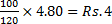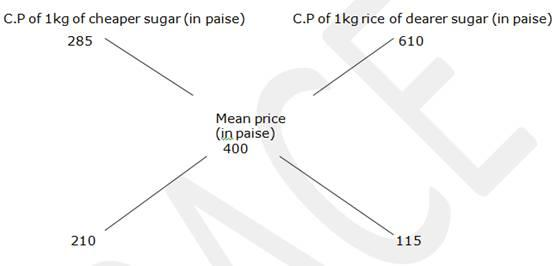=210:115  =42:23

If cheaper sugar is 42

If dearer one is 23

If cheaper sugar is 126 kg, dearer one=(23/42)*126=69kg

4) A showroom owner sells a leather jacket for Rs. X and claims to make a profit of 10%. He plans to have a stall in the trade fair and marks the same jacket at Rs.2X. At the stall, he allows a discount of 20%. What will be the percentage profit that he will make at the trade fair?

a) 80%

b) 60%

c) 76%

d) 84%

c)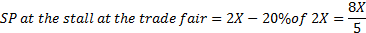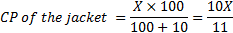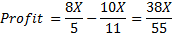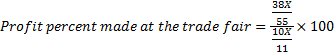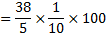76%

5) In a polling booth, total number of voters is 1575, out of which 0.4 part are male voters. If a candidate gets 0.6 part of male votes and 0.4 part of female votes, then find how many votes did the candidate get?

a) 945

b) 756

c) 378

d) 630

e) 543

b)

Number of male Voters = (4/10) of 1575

Number of male voters = 630

Number of female voters = 1575-630=945

Now, total votes that candidate got

=(6/10)*630+(4/10)*945

=378+378

=756

6) In a college election between two rivals, a candidate who got 40% of the total votes polled, was defeated by his rival by 200 votes. The total number of votes polled was

a) 800

b) 1000

c) 1200

d) 600

e) None of these

b)

Let total number of votes polled be x.

Then, votes polled by other candidate

=(100-40)%of x= 60%of x

Now 60% of x-40% of x = 200

7) A number is increased by 12% and then reduced by 10%. After these operations, the number:

a) Does not change

b) Decreases by 0.8%

c) Increase by 8%

d) Increase by 0.8%

e) None of these

d)

Let the original number be 100

Then, the number = 100 * 1.12*0.90=100.8

The number increases by 0.8%

increases 0.8%

8) Akash scored 72 marks in subject A. He scored 56% marks in subject B and X marks in subject C. Maximum marks in each subject were 150. The overall percentage marks obtained by Akash in all the three subjects together were 54%. How many marks did he score in subject C?

a) 84

b) 87

c) 79

d) 73

e) None of these

b)

9) Anuradha deposited 14% of the initial amount to his locker. And again after some time he deposited 45% of the increased amount. Now the amount becomes Rs 16530. How much was the initial amount?

a) Rs.10500

b) Rs.10000

c) Rs.9500

d) Rs.9000

e) None of these

b)

Let initial amount=100

Now, total amount =100+14+45/100×114=165.3

165.3  16530

16530/1653

100 100×100=10000Rs

10) In an examination it is required to get 65% of the aggregate marks to pass. A student gets 684 marks and is declared failed by 8% marks. What are the maximum aggregate marks a student can get?

a) 650

b) 1200

c) 1050

d) Cannot be determined

e) None of the above